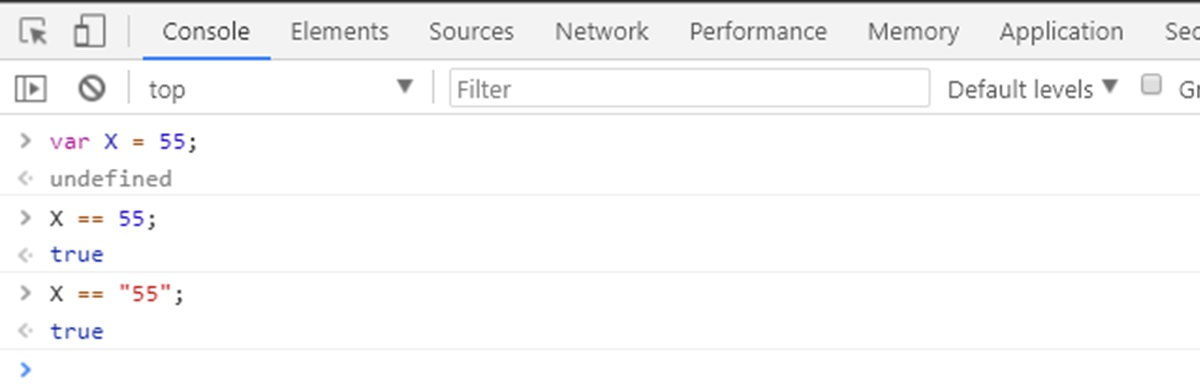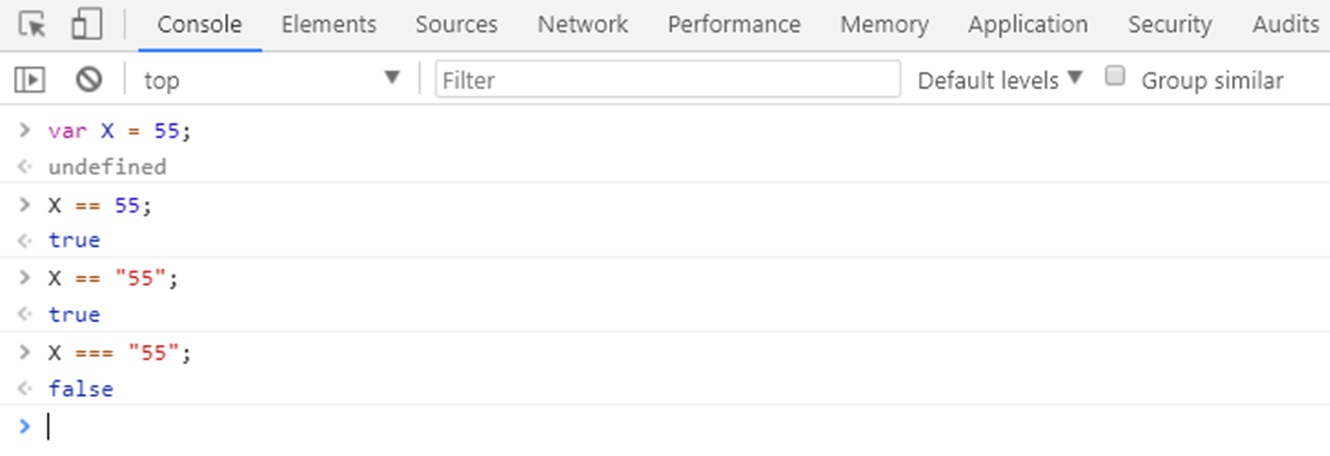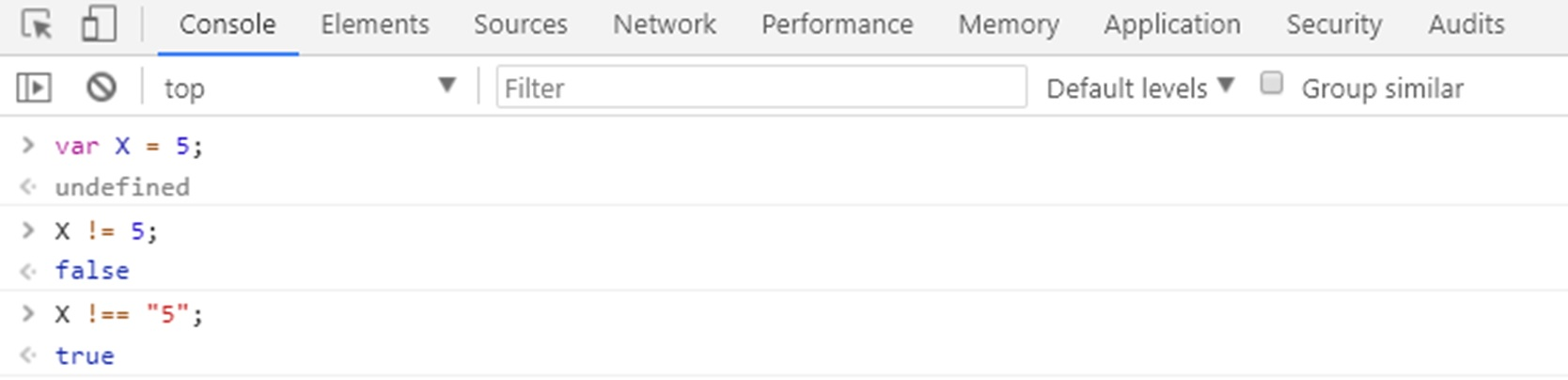# Lesson 8 Equality and Inequality Operators In Javascript

In this lesson, we are learning more about Boolean logic, and we will also discuss what equality and inequality operators in JavaScript and we will show you with examples how you can perform equality and inequality operators.

## Equality and Inequality Operators

There are two ways to check equality and inequality in JavaScript Boolean.

### Equality

There are basically two way to perform equality in JavaScript:

Double equal sign (==) perform some conversion before comparing the actual value, while tripe equal sign (===) will directly compare the value without doing any conversion.

There is basically two way to perform equality in JavaScript: Double equal sign (==) perform some conversion before comparing the actual value, while tripe equal sign (===) will directly compare the value without doing any conversion.

Let suppose we have an ‘X’ whose value is 5 (X=5), now in JavaScript, it is true if we write X==5 or X== “5” (here JavaScript convert String into value) or X===5, while it is false, if we perform X===“5” it is because here “5” treated as a string.

X==5 TRUE
X== “5”   TRUE
X===5   TRUE
X===“5”   FALSE

When we use double equal sign (== ), it performs type coercion.
It means that it takes two numbers or strings or two variables and then tries to make it into a similar type so that it can compare.

So here is an example, where we have declared a variable X with the value 55.

## JavaScript Code

``````var X = 55;
X == 55;
X == "55";
``````You can see here in above result, when we performing X == 55 or X == “55” where 55 in quotes is string but it perform some conversion first convert the value into similar type, in this case, it converting type from string to number and then it showing output that its true.

And here is another example where we performing comparison rule with three equals sign like X === “55”. Here 55 treated as a string.You can see in above output three equal sign (===) does not care about type version as well as the value and that’s why the output is false.

NOTE: you should always use === because it is much safer, it’s much more specific.

## Inequality

There is also two way to check inequality in JavaScript:

### Assume X = 5

Operator Name Example
X != 5 X is not equal to 5 False
X !== “5” X is not equal to string 5 TRUE

Let suppose we have an ‘X’ whose value is 5 (X=5), now in JavaScript, it is False if we write X != 5 it is because in real X value is 5 or X !== “5”, here 5 in quotation treats as string which is obviously not going to number 5 that’s why this statement is true.In this lesson, we have learned more about Boolean logic, and we have discussed what equality and inequality operators in JavaScript and perform some example of equality and inequality operators which show how we make decisions in our JavaScript.